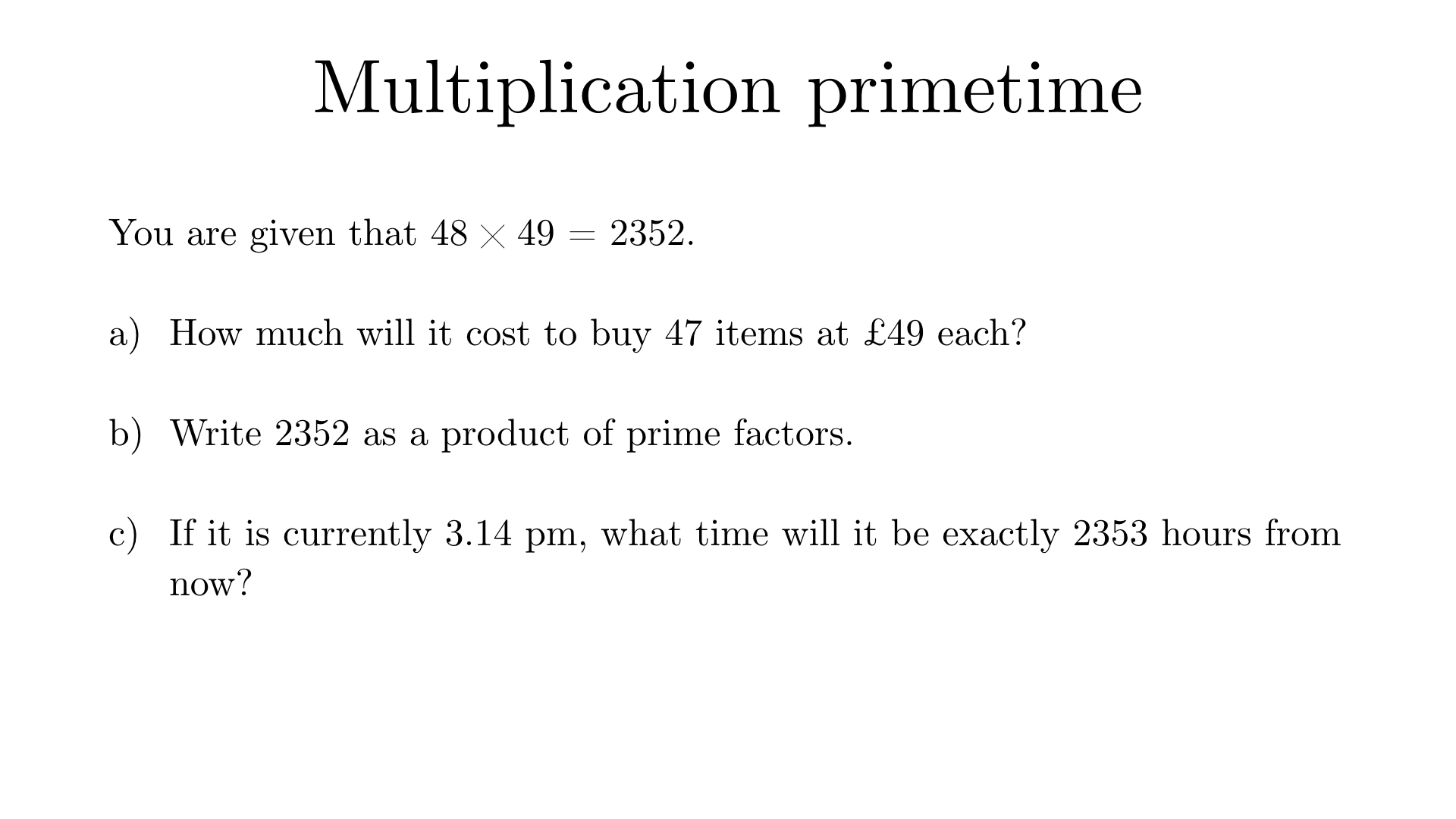Solution to (a)
$$47 \times 49 = 48 \times 49 – 1 \times 49$$$$= 2352 – 49 = 2303$$
Solution to (b)
$$48 = 2^4 \times 3$$ and $$49 = 7^2$$ so $$2352 = 2^4 \times 3 \times 7^2$$
Solution to (c)
Since $$2352$$ is a multiple of $$48$$, it is also a multiple of $$24$$. Therefore the remainder when $$2353$$ is divided by $$24$$ is $$1$$, so the time will be $$4.14 \text{pm}$$.